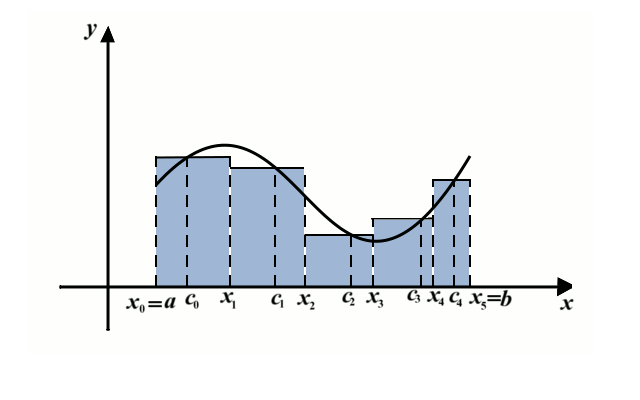# Definite Integrals. Fundamental Theorem of Calculus

## Integral Sum

The definite integral can be understood as the area under the graph of the function. In order to define the integral properly, we need the concept of integral sum.

Assume that $$f$$ is a continuous function defined on the interval $$[a,b]$$. A partition of the interval $$[a,b]$$ is a sequence of points $$x_0$$, $$x_1$$, $$\dots$$, $$x_n$$ such that $$x_0=a$$, $$x_n=b$$, and $$x_0\leq x_1\leq x_2\leq\cdots\leq x_n$$. Let $$c_0$$ be any point from the interval $$[x_0,x_1]$$, $$c_1\in[x_1,x_2]$$, $$\dots$$, $$c_{n-1}\in [x_{n-1},x_n]$$. An integral sum (also called the Riemann sum) corresponding to the partition $$x_0$$, $$x_1$$, $$\dots$$, $$x_n$$, and the numbers $$c_0$$, $$c_1$$, $$\dots$$, $$c_{n-1}$$ is defined as $S(f,x_0,x_1,\dots, x_n,c_0,\dots, c_{n-1})=f(c_0)\cdot (x_1-x_0)+f(c_1)\cdot (x_2-x_1)+f(c_2)\cdot (x_3-x_2)+\cdots+ f(c_{n-1})\cdot (x_n-x_{n-1}).$

This quantity $$S$$ depends on the choice $$c_0$$, $$c_1$$, $$\dots$$, $$c_{n-1}$$, and the partition $$x_0$$, $$x_1$$, $$\dots$$, $$x_n$$. The surface area of the shaded region in the picture below is an example for the integral sum with $$n=5$$.The maximum of the numbers $$\{|x_1-x_0|, |x_2-x_1|,\dots, |x_n-x_{n-1}|\}$$, usually denoted by $$\delta$$ is called the diameter of the partition. The limit of the integral sums as the diameter of the partition approaches $$0$$ is called the integral of the function $$f$$ on the interval $$[a,b]$$ and is denoted by $$\int_a^b f(x)\,dx$$. Here is the precise definition:

Definition (Definite integral)

A function $$f:[a,b]\to\mathbb R$$ is integrable and its integral over the segment $$[a,b]$$ is equal to a real number $$I\in\mathbb R$$ if for each $$\varepsilon> 0$$ there exists $$\delta> 0$$ such that for every partition $$a=x_0\leq x_1\leq\dots\leq x_n=b$$ with $$\delta(x_0,\dots, x_n)< \delta$$ we have $|S(f,x_0,x_1,\dots, x_n,c_0,\dots, c_n)-I|< \varepsilon$ for every choice of $$c_0\in[x_0,x_1]$$, $$c_1\in[x_1,x_2]$$, $$\cdots$$, $$c_{n-1}\in[x_{n-1},x_n]$$.

The above-described limit does not exist for all functions $$f$$, but if such a limit exists, the function is called integrable/ All continuous functions are integrable. Also, all bounded functions that have countably many discontinuities are integrable as well. The class of integrable functions is surprisingly large, but our aim is not to go into details at this point.

## Fundamental theorem of calculus

The fundamental theorem of calculus makes a connection between antiderivatives and definite integrals. The first theorem that we will present shows that the definite integral $$\int_a^xf(t)\,dt$$ is the anti-derivative of a continuous function $$f$$. This theorem is sometimes referred to as First fundamental theorem of calculus. Its name is actually differentiation theorem.

Theorem (Differentiation Theorem)

Assume that $$f$$ is a continuous function on $$[a,b]$$. Let $$x\in(a,b)$$, and let $$F(x)=\int_a^xf(t)\,dt$$. Then for each $$x\in(a,b)$$, $$F$$ is a differentiable function and $$x\in(a,b)$$ and \begin{eqnarray*}F’(x)=f(x).\end{eqnarray*}

Here we will present the formulation and the proof of the fundamental theorem of calculus. This theorem is also known as Newton-Leibnitz formula.

Theorem (Fundamental Theorem of Calculus)

If $$f$$ is a continuous function on $$[a,b]$$ and $$F$$ its antiderivative, then $\int_a^b f(x)\,dx=F(b)-F(a).$

Remark. There are many generalizations of this theorem that can relax our assumptions of continuity of $$f$$.

## Examples

In evaluating the integrals $$\displaystyle \int_a^bf(x)\,dx$$ one needs to find an antiderivative $$F$$ of the function $$f$$, and to calculate $$F(b)-F(a)$$. The notation $$\displaystyle \left.F(x)\right|_{a}^b$$ is often used to replace the expression $$F(b)-F(a)$$. Sometimes we also use $$\displaystyle \left.F(x)\right|_{x=a}^{x=b}$$ for the same purpose especially if the function $$F$$ depends on some other parameters and we want to avoid any possible ambiguity.

Example 1.

Evaluate the integral $$\displaystyle \int_0^6x^2\,dx$$.

Example 2.

Evaluate the integral $$\displaystyle \int_0^{\frac{\pi}2}\cos x\,dx$$.

Example 3.

Evaluate the integral $$\displaystyle \int_1^e\ln x\,dx$$.

2005-2021 IMOmath.com | imomath"at"gmail.com | Math rendered by MathJax